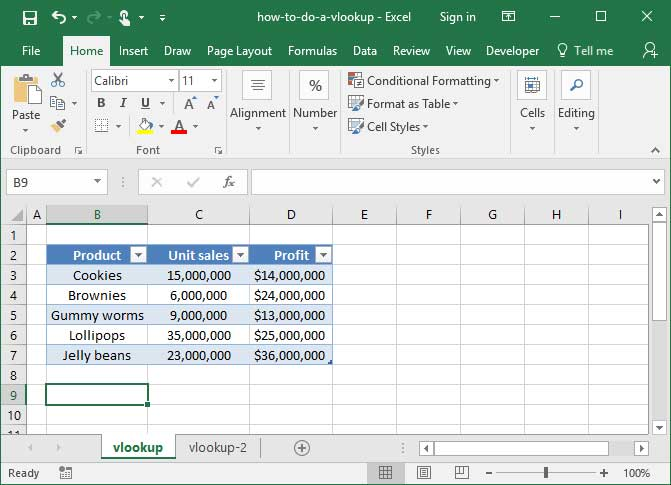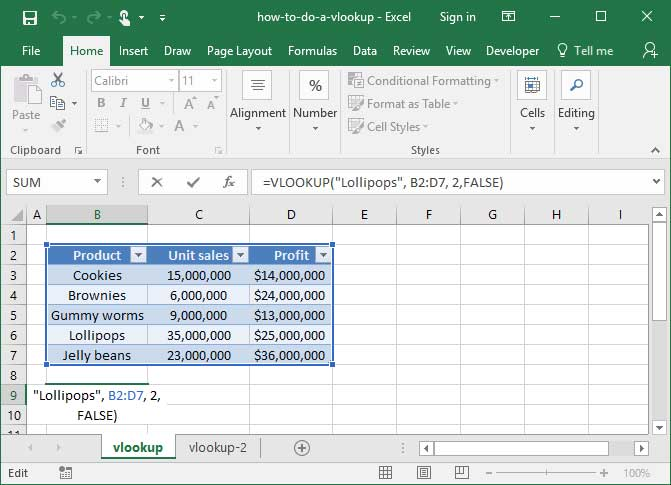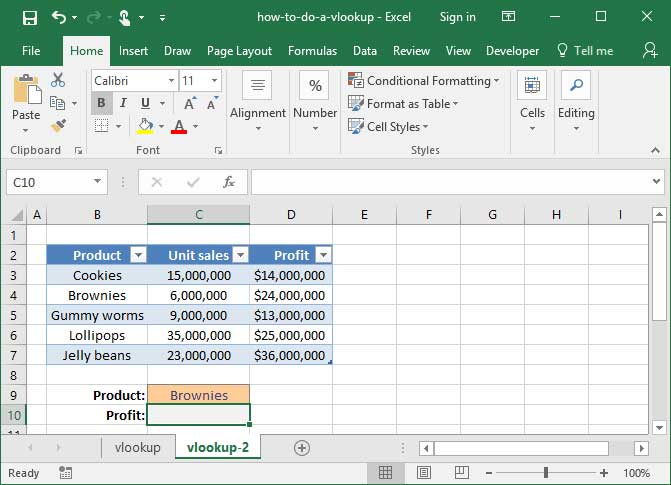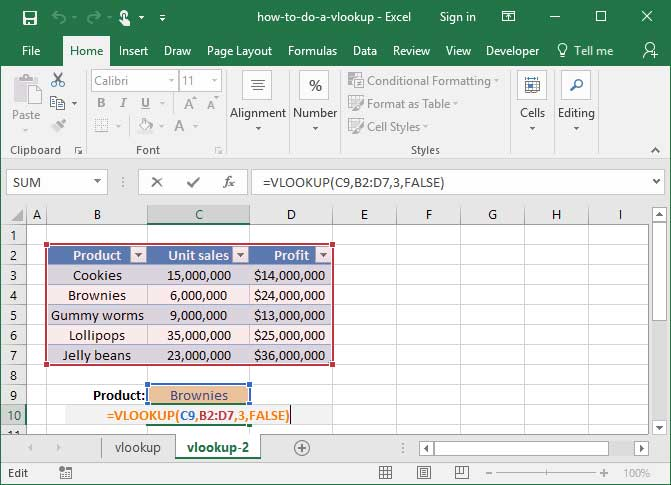Thanks for checking out a trial of the Advanced Excel Functions course!
Here's a sample lesson to start us off.

# VLOOKUP

VLOOKUP, one of Excel's most powerful functions, is used to look up data from a foreign table. Learn how to do a VLOOKUP in this handy tutorial!

### VLOOKUP

In this lesson, we’re going to learn about one of Excel’s most common — and complex — functions: VLOOKUP. If you’ve been using Excel for a while, you’ve almost undoubtedly heard of VLOOKUP. It’s a common tool used in a huge number of spreadsheets around the world, and learning it is an important stepping stone to automating a lot of your day-to-day tasks in Excel.

Let’s start with an example of what the function can do. In short, VLOOKUP — which is short for “vertical lookup” — allows us to look up a given value from a vertically-oriented table of data. We’ve created an example workbook here so that you can see it in action. Our sheet lists 2016 SnackWorld sales numbers for a number of different product categories. We’ve created a VLOOKUP function that pulls the proper sales number if we enter the name of a given product category in this “Product” box up top. Check it out — when I enter “Cakes” into the box, our VLOOKUP function updates the “Sales” total to \$1,646,500 — the sales total for the Cakes category. When I change the product category in our input box to Brownies, our Sales total changes accordingly.

You might be wondering why we wouldn’t just use Excel’s “Find” function if we wanted to find the Sales total for Cakes. We could pretty easily search for the word “Cakes” in our spreadsheet and find the sales total that way instead of writing a complicated formula.

First, we might be dealing with a mountain of data for which using Excel’s “Find” function would be too time-consuming. In this other sheet, for example, we’ve used the VLOOKUP function to pull in the categories of various products from an external table. There are a lot of orders, and looking up each of the product categories manually would have been way too time-consuming.

Second, the ability to dynamically update cells based on user input — like what we’ve done in our first sheet here — will become important down the line as we begin to develop more complex spreadsheets and models. We’ll investigate this further in later lessons.

Alright — so now that we know what VLOOKUP is, how do we use it? Getting a handle on this function is a bit complicated, but we’ll walk through it step by step to make it easy:

VLOOKUP takes four arguments: A value to look up, an array of cells in which to look up that value, a “column index number” that tells Excel how many columns into the array to look for a result, and a ‘range lookup’ argument that tells Excel whether to look for an exact match to your specified lookup term, or the closest match found in your lookup table. Let’s try it out using our sales data table from earlier. Recall that when using VLOOKUP, the table of data we use must be vertically-oriented — meaning that column headers appear along the top, and the value we want to look up appears along the side. We’ve also got to make sure that the value we want to look up — in this case, the name of a product — appears in the first column of the table in question.

Let’s get started. First, we’ll feed VLOOKUP a value to look up. In this case, let’s use the string, “Brownies”. Then, we’ll give it an array in which to look up our string. It’s important that when specifying the array here, we use our entire table rather than just the column in which our lookup value appears. In this case, our entire table spans from cells B2 to D17.

Next, we’ll give VLOOKUP a “column index number”. This number tells the function how many columns in to our data to look for a result. To use VLOOKUP, we literally have to count the number of columns of data we have.

In this case, we want to pull the number from the “Sales” column — which is one, two columns in to our data set. So, we’ll provide VLOOKUP with a column index number of 2.

Finally, we need to provide VLOOKUP with a ‘range lookup’ argument. This argument has to be either the word TRUE, or the word FALSE. If the ‘range lookup’ is TRUE, VLOOKUP will assume that your input array is sorted alphabetically — and will find the closest match to your specified lookup term. If ‘range lookup’ is FALSE, VLOOKUP won’t assume anything about the sorting of your input array — and it’ll only find an exact match with the term you specify.

When we use VLOOKUPs, we almost always set the ‘range lookup’ to FALSE. That way, we don’t have to worry about sorting our input table, and we know our VLOOKUP will only succeed if there is an exact match with our input term.

After pressing enter, our formula is complete. Note that VLOOKUP is pulling the correct value from the Sales column for the Brownies category!

Let’s make some changes in the formula to get an even better handle on how VLOOKUP works. First, we’ll change the lookup string to another product category, like “Cakes”. Note that when we change the string and press enter, our VLOOKUP formula updates its output to reflect the sales numbers for the Cakes category.

Second, we’ll try pulling lookup data from a different column. Let’s say that we wanted to pull data from our “Units sold” column rather than our “Sales total” column. To do so, we’d just have to update our column index number to three, since the “units sold” column is one, two, three columns into our input array. After making the update, our VLOOKUP automatically changes to reflect the proper number pulled from the “units sold” column.

Finally, we’ll wire our VLOOKUP function up to a dynamic input cell using cell references rather than manually coding our product name into the formula. Let’s enter a product name in cell G2, then replace the producy name in our VLOOKUP formula with a reference to cell G2. The output of our VLOOKUP formula automatically updates. And, like magic, our VLOOKUP will pull new data if we change the product name in cell G2. Note that we do need to provide VLOOKUP with a valid product name; an invalid product name will result in a formula error.

Now that our VLOOKUP is complete, I’ve got to warn you about one important caveat to this otherwise very useful function. Recall that in constructing our VLOOKUP, we had to manually count how many columns into our data table for the function to go to pull results. That’s fine and well, but it becomes a problem if we insert a new column into our data set. Check it out — when I put in a new column of data, our VLOOKUP no longer returns the proper results. That’s because it’s looking 3 columns into our data set for an answer — and the target column, “Units sold” is no longer 3 columns into our data set. After inserting this row, we’ll have to update our VLOOKUP function with a new ‘column index number’ to fix it.

Moral of the story: when using VLOOKUPs, always be careful when inserting columns into your data set, as it may have unforeseen consequences. That’s why I almost always recommend that Excel users replace their VLOOKUPs with a function called INDEX MATCH — which contains much of the same functionality, without this important drawback. We’ll explore INDEX MATCH in a later video in this module.

That’s it — you now know how to put together a VLOOKUP function in Excel! Take a look at the exercises bundled with this video to get more practice with this versatile function.

If you've spent time with Excel, you've probably heard of VLOOKUP. It's one of the most popular and useful functions you can use on a spreadsheet, and is found in most workbooks that venture beyond simple mathematical functions like SUM and AVERAGE. What is this ubiquitous function, and how can it help you save time?

In short, VLOOKUP allows you to look up a value from a separate table based on any index you choose. For example: You could dynamically look up the price of a specific item based on a price list; you could reference sales for a particular month based on a list of monthly revenue numbers; or, you could look up the name of a user based on their e-mail address.

That's a lot to take in, but things will become much clearer once we take a look at some examples below.

## Defining the problem

Take a look at the simple spreadsheet below, which lists SnackWorld's January sales of various types of candy.Let's say someone asked us how many Lollipops we sold in January. To answer their question, we'd have to open up the table, scan the rows until we find the word Lollipops, and then tell them the number that sits next to it: 35,000,000.

That's easy to do when we have a small table like the one in the example above. But you can imagine it being very difficult if the table is large. It'd be a real pain to have to sift through hundreds of rows of data each time we want to look up the sales for a particular product.

That's where VLOOKUP comes in. We can use this function to dynamically look up the unit sales of a particular item from the table, based on its name alone.

## Using VLOOKUP

Here's the syntax of the VLOOKUP formula:

`=VLOOKUP(lookup_value, table_array, col_index_num, range_lookup)`

First, VLOOKUP takes a lookup_value argument, which is a string containing the phrase that we'd like to look up — like "Lollipops", or "Cotton Candy".

Second, the function takes a table_array argument. This is the table from which we'll be looking up our values, and is specified as a cell range like B2:D7.

Third, VLOOKUP needs to know what column to pull data from. You tell it using the col_index_num argument. This argument is a number that tells VLOOKUP how many columns it should read in the table to access the data we care about. An argument of 1 will pull from the first column in the table you specify. Likewise, an argument of 3 will pull from the third column.

Finally, VLOOKUP takes a range_lookup argument. The range_lookup can be either TRUE or FALSE. If it's TRUE — or if it is omitted — VLOOKUP will assume that our input table_array is sorted in ascending order, and will find the nearest table value that is still less than the lookup_value specified. If range_lookup is set to FALSE, VLOOKUP will only return an exact match with the specified lookup term.

We almost always use FALSE as the value for our range_lookup argument, because most of the time we look for an exact match with our specified lookup term. For now, assume that you should always use FALSE as that argument, and we'll take a look at some examples later on of when you might want to mix things up!

Here's a complete example of the whole formula together:`=VLOOKUP("Lollipops", B2:D7, 2, FALSE)Output: 35,000,000`

What's happening here? First, VLOOKUP looks at our table_array argument, which it sees is the table B2:D7.

Next, it looks for the lookup_value argument — which in this case is "Lollipops" — in the first column of that table. Note that our lookup value, "Lollipops", is in Column B, the first column within our table_range of B2:D7 — a critical condition for this formula to work.

Now, Excel knows that we want to pull some data from Row 6, since that's where it found the word "Lollipops" in the first column of the table we specified. To finish, it needs to know which of the other columns we want to pull data from.

So, it follows the table across col_index_num columns to find the value it's looking up. In this case, we've set our col_index_num to 2, because the Unit sales column is the second column in to the table we've specified. Therefore, the output value is 35,000,000. If we wanted VLOOKUP to return the Profit instead, we would set the col_index_num to 3, because the Profit column is the third column in to the table we've specified.

Note that our range_lookup argument is set to FALSE, so VLOOKUP will only pull an exact match to our specified lookup term.

## Another example

Let's check out another, slightly more complicated example of VLOOKUP. This time, we want to create a dynamic set of input fields that let us look up profit by product category automatically:We want to write a formula in the empty grey box that will look up the Profit for whatever Product we choose. How can we do that?

The answer: We'll use dynamic cell references within a VLOOKUP formula to reference our Product input cell. Check out the following solution:`=VLOOKUP(C9, B2:D7, 3, FALSE)Output: \$24,000,000`

Let's break down what's happening here: First, VLOOKUP checks out our table_array argument, where it finds the range B2:D7. To find out which row of that table to pull, it looks to the lookup_value argument, where it finds the cell C9. Within cell C9 is the string "Brownies", so Excel uses that to identify the row we care about. Finally, VLOOKUP examines the col_index_num argument to figure out how many columns it should count in to pull data. We've told it to count in 3 columns, leading it to the Profit heading and a final output value of \$24,000,000.

Now you can see how powerful VLOOKUP is — it will allow you to look up any value, in any column, off of any table, with dynamic inputs that help identify the row you care about. There are infinite applications for this capability, and we hope that this tutorial helps you find some great ones!

Advanced Excel Functions: 0 of 37 lessons completed
0%